# 10.5 Graphing quadratic equations  (Page 5/15)

 Page 5 / 15

Finding the y -intercept by substituting $x=0$ into the equation is easy, isn’t it? But we needed to use the Quadratic Formula to find the x -intercepts in [link] . We will use the Quadratic Formula again in the next example.

Graph $y=2{x}^{2}-4x-3$ .

## Solution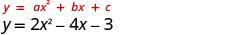The equation y has one side. Since a is 2, the parabola opens upward.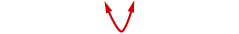To find the axis of symmetry, find $x=-\frac{b}{2a}$ .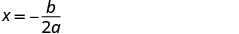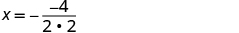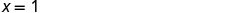The axis of symmetry is $x=1$ . The vertex on the line $x=1.$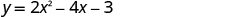Find y when $x=1$ .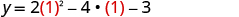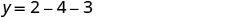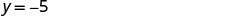The vertex is $\left(1,\text{−}5\right)$ . The y -intercept occurs when $x=0.$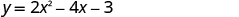Substitute $x=0.$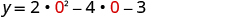Simplify.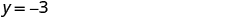The y- intercept is $\left(0,-3\right)$ . The point $\left(0,-3\right)$ is one unit to the left of the line of symmetry. The point one unit to the right of the line of symmetry is $\left(2,-3\right)$ Point symmetric to the y- intercept is $\left(2,-3\right).$ The x -intercept occurs when $y=0$ .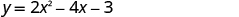Substitute $y=0$ .Use the Quadratic Formula.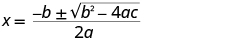Substitute in the values of a, b, c.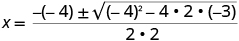Simplify.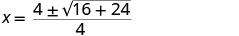Simplify inside the radical.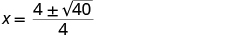Simplify the radical.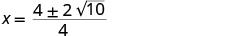Factor the GCF.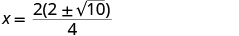Remove common factors.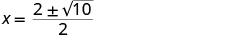Write as two equations.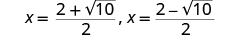Approximate the values.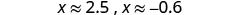The approximate values of the x- intercepts are $\left(2.5,0\right)$ and $\left(-0.6,0\right)$ . Graph the parabola using the points found.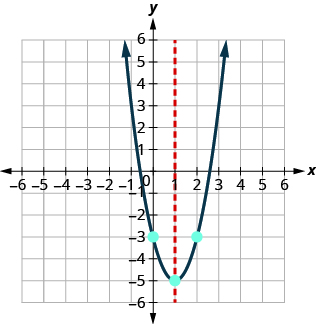Graph the parabola $y=5{x}^{2}+10x+3.$

$y\text{:}\phantom{\rule{0.2em}{0ex}}\left(0,3\right);\phantom{\rule{0.2em}{0ex}}x\text{:}\phantom{\rule{0.2em}{0ex}}\left(-1.6,0\right),\left(-0.4,0\right);$
axis: $x=-1;\phantom{\rule{0.2em}{0ex}}\text{vertex:}\phantom{\rule{0.2em}{0ex}}\left(-1,-2\right)$ ;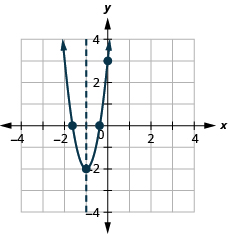Graph the parabola $y=-3{x}^{2}-6x+5.$

$y\text{:}\phantom{\rule{0.2em}{0ex}}\left(0,5\right);\phantom{\rule{0.2em}{0ex}}x\text{:}\phantom{\rule{0.2em}{0ex}}\left(0.6,0\right),\left(-2.6,0\right);$
axis: $x=-1;\phantom{\rule{0.2em}{0ex}}\text{vertex:}\phantom{\rule{0.2em}{0ex}}\left(-1,8\right)$ ;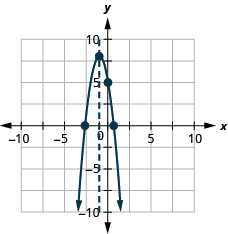## Solve maximum and minimum applications

Knowing that the vertex    of a parabola is the lowest or highest point of the parabola gives us an easy way to determine the minimum or maximum value of a quadratic equation. The y -coordinate of the vertex is the minimum y -value of a parabola that opens upward. It is the maximum y -value of a parabola that opens downward. See [link] .

## Minimum or maximum values of a quadratic equation

The y -coordinate of the vertex of the graph of a quadratic equation is the

• minimum value of the quadratic equation if the parabola opens upward.
• maximum value of the quadratic equation if the parabola opens downward.

Find the minimum value of the quadratic equation $y={x}^{2}+2x-8$ .

## Solution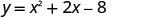Since a is positive, the parabola opens upward. The quadratic equation has a minimum. Find the axis of symmetry.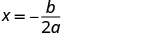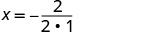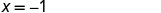The axis of symmetry is $x=-1$ . The vertex is on the line $x=-1.$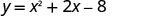Find y when $x=-1.$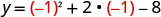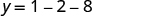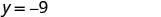The vertex is $\left(-1,-9\right)$ . Since the parabola has a minimum, the y- coordinate of the vertex is the minimum y- value of the quadratic equation. The minimum value of the quadratic is $-9$ and it occurs when $x=-1$ . Show the graph to verify the result.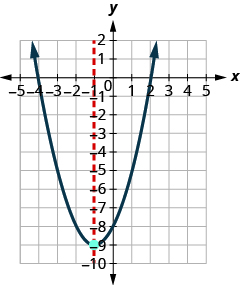Find the maximum or minimum value of the quadratic equation $y={x}^{2}-8x+12$ .

The minimum value is $-4$ when $x=4$ .

Find the maximum or minimum value of the quadratic equation $y=-4{x}^{2}+16x-11$ .

The maximum value is 5 when $x=2$ .

We have used the formula

$h=-16{t}^{2}+{v}_{0}t+{h}_{0}$

to calculate the height in feet, $h$ , of an object shot upwards into the air with initial velocity, ${v}_{0}$ , after $t$ seconds.

This formula is a quadratic equation in the variable $t$ , so its graph is a parabola. By solving for the coordinates of the vertex, we can find how long it will take the object to reach its maximum height. Then, we can calculate the maximum height.

The quadratic equation $h=-16{t}^{2}+{v}_{0}t+{h}_{0}$ models the height of a volleyball hit straight upwards with velocity 176 feet per second from a height of 4 feet.

1. How many seconds will it take the volleyball to reach its maximum height?
2. Find the maximum height of the volleyball.

## Solution

$h=-16{t}^{2}+176t+4$

Since a is negative, the parabola opens downward.

The quadratic equation has a maximum.

1. $\begin{array}{cccc}\text{Find the axis of symmetry.}\hfill & & & \phantom{\rule{4em}{0ex}}\begin{array}{c}t=-\frac{b}{2a}\hfill \\ t=-\frac{176}{2\left(-16\right)}\hfill \\ t=5.5\hfill \end{array}\hfill \\ & & & \phantom{\rule{4em}{0ex}}\text{The axis of symmetry is}\phantom{\rule{0.2em}{0ex}}t=5.5.\hfill \\ \text{The vertex is on the line}\phantom{\rule{0.2em}{0ex}}t=5.5.\hfill & & & \phantom{\rule{4em}{0ex}}\text{The maximum occurs when}\phantom{\rule{0.2em}{0ex}}t=5.5\phantom{\rule{0.2em}{0ex}}\text{seconds.}\hfill \end{array}$

2.  Find h when $t=5.5$ .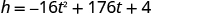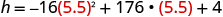Use a calculator to simplify.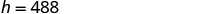The vertex is $\left(5.5,488\right)$ . Since the parabola has a maximum, the h- coordinate of the vertex is the maximum y -value of the quadratic equation. The maximum value of the quadratic is 488 feet and it occurs when $t=5.5$ seconds.

He charges $125 per job. His monthly expenses are$1,600. How many jobs must he work in order to make a profit of at least $2,400? Alicia Reply at least 20 Ayla what are the steps? Alicia what is algebra Azhar Reply repeated addition and subtraction of the order of operations. i love algebra I'm obsessed. Shemiah hi Krekar One-fourth of the candies in a bag of M&M’s are red. If there are 23 red candies, how many candies are in the bag? Leanna Reply rectangular field solutions Navin Reply What is this? Donna the proudact of 3x^3-5×^2+3 and 2x^2+5x-4 in z7[x]/ is anas Reply ? Choli a rock is thrown directly upward with an initial velocity of 96feet per second from a cliff 190 feet above a beach. The hight of tha rock above the beach after t second is given by the equation h=_16t^2+96t+190 Usman Stella bought a dinette set on sale for$725. The original price was $1,299. To the nearest tenth of a percent, what was the rate of discount? Manhwa Reply 44.19% Scott 40.22% Terence 44.2% Orlando I don't know Donna if you want the discounted price subtract$725 from $1299. then divide the answer by$1299. you get 0.4419... but as percent you get 44.19... but to the nearest tenth... round .19 to .2 and you get 44.2%
Orlando
you could also just divide $725/$1299 and then subtract it from 1. then you get the same answer.
Orlando
p mulripied-5 and add 30 to it
Tausif
Tausif
Can you explain further
p mulripied-5 and add to 30
Tausif
How do you find divisible numbers without a calculator?
TAKE OFF THE LAST DIGIT AND MULTIPLY IT 9. SUBTRACT IT THE DIGITS YOU HAVE LEFT. IF THE ANSWER DIVIDES BY 13(OR IS ZERO), THEN YOUR ORIGINAL NUMBER WILL ALSO DIVIDE BY 13!IS DIVISIBLE BY 13
BAINAMA
When she graduates college, Linda will owe $43,000 in student loans. The interest rate on the federal loans is 4.5% and the rate on the private bank loans is 2%. The total interest she owes for one year was$1,585. What is the amount of each loan?
Sean took the bus from Seattle to Boise, a distance of 506 miles. If the trip took 7 2/3 hours, what was the speed of the bus?
66miles/hour
snigdha
How did you work it out?
Esther
s=mi/hr 2/3~0.67 s=506mi/7.67hr = ~66 mi/hr
Orlando
hello, I have algebra phobia. Subtracting negative numbers always seem to get me confused.
what do you need help in?
Felix
Heather
look at the numbers if they have different signs, it's like subtracting....but you keep the sign of the largest number...
Felix
for example.... -19 + 7.... different signs...subtract.... 12 keep the sign of the "largest" number 19 is bigger than 7.... 19 has the negative sign... Therefore, -12 is your answer...
Felix
—12
Thanks Felix.l also get confused with signs.
Esther
Thank you for this
Shatey
ty
Graham
think about it like you lost $19 (-19), then found$7(+7). Totally you lost just $12 (-12) Annushka I used to struggle a lot with negative numbers and math in general what I typically do is look at it in terms of money I have -$5 in my account I then take out 5 more dollars how much do I have in my account well-\$10 ... I also for a long time would draw it out on a number line to visualize it
Meg
practicing with smaller numbers to understand then working with larger numbers helps too and the song/rhyme same sign add and keep opposite signs subtract keep the sign of the bigger # then you'll be exact
Meg
Bruce drives his car for his job. The equation R=0.575m+42 models the relation between the amount in dollars, R, that he is reimbursed and the number of miles, m, he drives in one day. Find the amount Bruce is reimbursed on a day when he drives 220 miles
168.50=R
Heather
john is 5years older than wanjiru.the sum of their years is27years.what is the age of each
46
mustee
j 17 w 11
Joseph
john is 16. wanjiru is 11.
Felix
27-5=22 22÷2=11 11+5=16
Joyce
I don't see where the answers are.
EdBy Candice ButtsBy Nick SwainByBy Qqq QqqBy OpenStaxBy David CoreyBy Sheila LopezBy Nicole DuquetteBy OpenStaxBy OpenStax